• 2019-09-30 15:09:33

调试广告出不来
太坑了，公司的网址有广告位，广告图片上传，
我这广告怎么刷新谷歌浏览器，清除缓存都没用，
最后找了好久是拦截广告插件的锅，调试页面广告怎么都不出来，但是元素还有图片地址都能正常访问，
乱找一通，还是这个插件，是的我又忘记了，大家记得调试时设置成在此网站上暂停。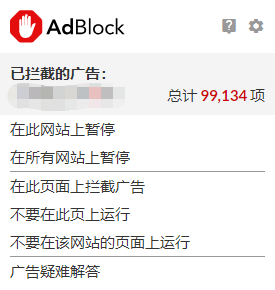调试
更多相关内容
• 广告拦截插件（扩展程序）安装到浏览器上，可以屏蔽掉大量的广告，让你享受纯净网页。 安装成功后，可以自行打开/关闭，进行对比，很明显的，启用该扩展程序后，可以看到被拦截广告的数量，网站上的垃圾广告会...

##### 安装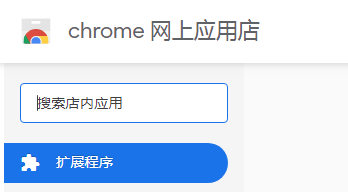点击安装 几分钟之后自动完成下载安装
有一个这样的标志就算完成安装了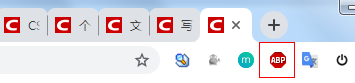##### 效果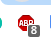有时候一些页面因为被拦截了广告会使页面显示异常，这时候可以关闭当前页面插件的广告拦截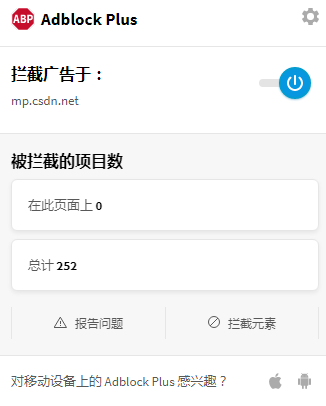展开全文chrome
• 最近做广告联盟的一个项目，在页面插入广告代码时被广告插件拦截了，这里简单的记录下解决方案。 方案一、代码直接写在页面上,不使用引入的方式。 以前直接引入是这样的: &amp;lt;script src=&...
• 最近做广告联盟的一个项目，在页面插入广告代码时被广告插件给拦截了，这里简单的记录下解决方案。

#### 方案一、代码直接写在页面上,不使用引入的方式。

• 以前直接引入是这样的:
<script src="http://xxxxxxx"> </script>

• 现在改成这样:
<script type="text/javascript">
//..................这里写src="http://xxxxxxx"那段js广告代码
</script>

• 测试了下这种是没有被插件拦截的(不过老板说代码量太大了，人家站长不会复制的，所以我放弃了这种);

#### 方案二、广告代码使用websocket方式返回，然后追加到页面上(我们使用的这个方案)。

• 我在网上查到目前广告插件对websocket拦截的不是那么严，也有的网站实现过了，所以自己也来试试。
• 首先来写段websocket代码，做服务端 webSocket.php:
<?php

error_reporting(E_ALL ^ E_NOTICE);
ob_implicit_flush();

//地址与接口，即创建socket时需要服务器的IP和端口
$sk=new Sock('192.168.0.111',9090); //对创建的socket循环进行监听，处理数据$sk->run();

/**
* 模拟post进行url请求
* @param string $url * @param array$post_data
*/
function request_post($url = '',$post_data = array()) {//url为必传  如果该地址不需要参数就不传
if (empty($url)) { return false; } if(!empty($post_data)){
$params = ''; foreach ($post_data as $k =>$v )
{
$params.= "$k=" . urlencode($v). "&" ; //$params.= "$k=" .$v. "&" ;
}
$params = substr($params,0,-1);
}
$ch = curl_init();//初始化curl curl_setopt($ch, CURLOPT_URL,$url);//抓取指定网页 curl_setopt($ch, CURLOPT_HEADER, 0);//设置header
curl_setopt($ch, CURLOPT_RETURNTRANSFER, 1);//要求结果为字符串且输出到屏幕上 //curl_setopt($ch, CURLOPT_POST, 1);//post提交方式  linux不传参数会返回400 Bad Request 错误所以暂时注释掉  ,windows不会
if(!empty($post_data))curl_setopt($ch, CURLOPT_POSTFIELDS, $params);$data = curl_exec($ch);//运行curl curl_close($ch);
return $data; } //下面是sock类 class Sock{ public$sockets; //socket的连接池，即client连接进来的socket标志
public $users; //所有client连接进来的信息，包括socket、client名字等 public$master;  //socket的resource，即前期初始化socket时返回的socket资源

private $sda=array(); //已接收的数据 private$slen=array();  //数据总长度
private $sjen=array(); //接收数据的长度 private$ar=array();    //加密key
private $n=array(); public function __construct($address, $port){ //创建socket并把保存socket资源在$this->master
$this->master=$this->WebSocket($address,$port);
error_log("Sock __construct ");
//创建socket连接池
$this->sockets=array($this->master);
}

//对创建的socket循环进行监听，处理数据
function run(){
//死循环，直到socket断开
while(true){
$changes=$this->sockets;
$write=NULL;$except=NULL;

/*
//这个函数是同时接受多个连接的关键，我的理解它是为了阻塞程序继续往下执行。
socket_select ($sockets,$write = NULL, $except = NULL, NULL);$sockets可以理解为一个数组，这个数组中存放的是文件描述符。当它有变化（就是有新消息到或者有客户端连接/断开）时，socket_select函数才会返回，继续往下执行。
$write是监听是否有客户端写数据，传入NULL是不关心是否有写变化。$except是$sockets里面要被排除的元素，传入NULL是”监听”全部。 最后一个参数是超时时间 如果为0：则立即结束 如果为n>1: 则最多在n秒后结束，如遇某一个连接有新动态，则提前返回 如果为null：如遇某一个连接有新动态，则返回 */ socket_select($changes,$write,$except,NULL);
foreach($changes as$sock){

//如果有新的client连接进来，则
if($sock==$this->master){

//接受一个socket连接
$client=socket_accept($this->master);

//给新连接进来的socket一个唯一的ID
$key=uniqid();$this->sockets[]=$client; //将新连接进来的socket存进连接池$this->users[$key]=array( 'socket'=>$client,  //记录新连接进来client的socket信息
'shou'=>false       //标志该socket资源没有完成握手
);
//否则1.为client断开socket连接，2.client发送信息
}else{
$len=0;$buffer='';
//读取该socket的信息，注意：第二个参数是引用传参即接收数据，第三个参数是接收数据的长度
do{
$l=socket_recv($sock,$buf,1000,0);$len+=$l;$buffer.=$buf; }while($l==1000);

//根据socket在user池里面查找相应的$k,即健ID$k=$this->search($sock);

//如果接收的信息长度小于7，则该client的socket为断开连接
if($len<7){ //给该client的socket进行断开操作，并在$this->sockets和$this->users里面进行删除$this->send2($k); continue; } //判断该socket是否已经握手 if(!$this->users[$k]['shou']){ //如果没有握手，则进行握手处理$this->woshou($k,$buffer);
}else{
//走到这里就是该client发送信息了，对接受到的信息进行uncode处理
$buffer =$this->uncode($buffer,$k);
if($buffer==false){ continue; } //error_log(" received buffer " .$buffer);
//如果不为空，则进行消息推送操作
$this->send($k,$buffer); } } } } } //指定关闭$k对应的socket
function close($k){ //断开相应socket socket_close($this->users[$k]['socket']); //删除相应的user信息 unset($this->users[$k]); //重新定义sockets连接池$this->sockets=array($this->master); foreach($this->users as $v){$this->sockets[]=$v['socket']; } //输出日志$this->e("key:$k close"); } //根据sock在users里面查找相应的$k
function search($sock){ foreach ($this->users as $k=>$v){
if($sock==$v['socket'])
return $k; } return false; } //传相应的IP与端口进行创建socket操作 function WebSocket($address,$port){$server = socket_create(AF_INET, SOCK_STREAM, SOL_TCP);
socket_set_option($server, SOL_SOCKET, SO_REUSEADDR, 1);//1表示接受所有的数据包 socket_bind($server, $address,$port);
socket_listen($server);$this->e('Server Started : '.date('Y-m-d H:i:s'));
$this->e('Listening on : '.$address.' port '.$port); return$server;
}

/*
* 函数说明：对client的请求进行回应，即握手操作
* @$k clien的socket对应的健，即每个用户有唯一$k并对应socket
* @$buffer 接收client请求的所有信息 */ function woshou($k,$buffer){ //截取Sec-WebSocket-Key的值并加密，其中$key后面的一部分258EAFA5-E914-47DA-95CA-C5AB0DC85B11字符串应该是固定的
$buf = substr($buffer,strpos($buffer,'Sec-WebSocket-Key:')+18);$key  = trim(substr($buf,0,strpos($buf,"\r\n")));
$new_key = base64_encode(sha1($key."258EAFA5-E914-47DA-95CA-C5AB0DC85B11",true));

//按照协议组合信息进行返回
$new_message = "HTTP/1.1 101 Switching Protocols\r\n";$new_message .= "Upgrade: websocket\r\n";
$new_message .= "Sec-WebSocket-Version: 13\r\n";$new_message .= "Connection: Upgrade\r\n";
$new_message .= "Sec-WebSocket-Accept: " .$new_key . "\r\n\r\n";
socket_write($this->users[$k]['socket'],$new_message,strlen($new_message));

//对已经握手的client做标志
$this->users[$k]['shou']=true;
return true;

}

//解码函数
function uncode($str,$key){
$mask = array();$data = '';
$msg = unpack('H*',$str);
$head = substr($msg,0,2);
if ($head == '81' && !isset($this->slen[$key])) {$len=substr($msg,2,2);$len=hexdec($len);//把十六进制的转换为十进制 if(substr($msg,2,2)=='fe'){
$len=substr($msg,4,4);
$len=hexdec($len);
$msg=substr($msg,4);
}else if(substr($msg,2,2)=='ff'){$len=substr($msg,4,16);$len=hexdec($len);$msg=substr($msg,16); }$mask[] = hexdec(substr($msg,4,2));$mask[] = hexdec(substr($msg,6,2));$mask[] = hexdec(substr($msg,8,2));$mask[] = hexdec(substr($msg,10,2));$s = 12;
$n=0; }else if($this->slen[$key] > 0){$len=$this->slen[$key];
$mask=$this->ar[$key];$n=$this->n[$key];
$s = 0; }$e = strlen($msg)-2; for ($i=$s;$i<= $e;$i+= 2) {
$data .= chr($mask[$n%4]^hexdec(substr($msg,$i,2)));$n++;
}
$dlen=strlen($data);

if($len > 255 &&$len > $dlen+intval($this->sjen[$key])){$this->ar[$key]=$mask;
$this->slen[$key]=$len;$this->sjen[$key]=$dlen+intval($this->sjen[$key]);
$this->sda[$key]=$this->sda[$key].$data;$this->n[$key]=$n;
return false;
}else{
unset($this->ar[$key],$this->slen[$key],$this->sjen[$key],$this->n[$key]);
$data=$this->sda[$key].$data;
unset($this->sda[$key]);
return $data; } } //与uncode相对 function code($msg){
$frame = array();$frame = '81';
$len = strlen($msg);
if($len < 126){$frame = $len<16?'0'.dechex($len):dechex($len); }else if($len < 65025){
$s=dechex($len);
$frame='7e'.str_repeat('0',4-strlen($s)).$s; }else{$s=dechex($len);$frame='7f'.str_repeat('0',16-strlen($s)).$s;
}
$frame =$this->ord_hex($msg);$data = implode('',$frame); return pack("H*",$data);
}

function ord_hex($data) {$msg = '';
$l = strlen($data);
for ($i= 0;$i<$l;$i++) {
$msg .= dechex(ord($data{$i})); } return$msg;
}

//用户加入或client发送信息
function send($k,$msg){
//将查询字符串解析到第二个参数变量中，以数组的形式保存如：parse_str("name=Bill&age=60",$arr) parse_str($msg,$g);$ar=array();

$fromDate = json_decode($msg);
error_log("json_decode(msg)[text] " . $fromDate->text); if($g['type']=='add'){
//第一次进入添加聊天名字，把姓名保存在相应的users里面
$this->users[$k]['name']=$g['ming'];$ar['type']='add';
$ar['name']=$g['ming'];
$key='all'; }else{ //发送信息行为，其中$g['key']表示面对大家还是个人，是前段传过来的信息
$ar['nrong']=$g['nr'];
$key=$g['key'];
}

$ar["requestUrl"] =$fromDate->text;
//推送信息
$this->send1($k,$ar,$key);
}

//对新加入的client推送已经在线的client
function getusers(){
$ar=array(); foreach($this->users as $k=>$v){
$ar[]=array('code'=>$k,'name'=>$v['name']); } return$ar;
}

//$k 发信息人的socketID$key接受人的 socketID ，根据这个socketID可以查找相应的client进行消息推送，即指定client进行发送
function send1($k,$ar,$key='all'){$ar['code1']=$key;$ar['code']=$k;$ar['time']=date('m-d H:i:s');
//对发送信息进行编码处理
$str =$this->code(json_encode($ar)); //面对大家即所有在线者发送信息 error_log("ar value " . json_encode($ar));
if($key=='all'){$users=$this->users; //如果是add表示新加的client if($ar['type']=='add'){
$ar['type']='madd';$ar['users']=$this->getusers(); //取出所有在线者，用于显示在在线用户列表中$str1 = $this->code(json_encode($ar)); //单独对新client进行编码处理，数据不一样
//对新client自己单独发送，因为有些数据是不一样的
socket_write($users[$k]['socket'],$str1,strlen($str1));
//上面已经对client自己单独发送的，后面就无需再次发送，故unset
unset($users[$k]);
}
//除了新client外，对其他client进行发送信息。数据量大时，就要考虑延时等问题了
foreach($users as$v){
socket_write($v['socket'],$str,strlen($str)); } }else{ //单独对个人发送信息，即双方聊天 //socket_write($this->users[$k]['socket'],$str,strlen($str)); error_log(" ar[requestUrl]" .$ar["requestUrl"]);
//发起请求得到广告
$responseBody = request_post($ar["requestUrl"], array());
$arrays = array();$arrays["responseBody"] = $responseBody;$str = $this->code(json_encode($arrays));
//error_log("responseBody ".$str["responseBody"]); socket_write($this->users[$k]['socket'],$str,strlen($str)); //socket_write($this->users[$key]['socket'],$str,strlen($str)); } } //用户退出向所用client推送信息 function send2($k){
$this->close($k);
$ar['type']='rmove';$ar['nrong']=$k;$this->send1(false,$ar,'all'); } //记录日志 function e($str){
//$path=dirname(__FILE__).'/log.txt';$str=$str."\n"; //error_log($str,3,$path); //编码处理 echo iconv('utf-8','gbk//IGNORE',$str);
}

}

echo "1";
?>


这代码是抄的,然后改了点东西。

• 服务端有了，再来写客户端的东西。
//这段代码需要引入jQuery
<script type="text/javascript">
//ws连接
var websocket;

/***
*zhouzhongqing
* 2018年6月1日14:50:02
* 发送消息
* */
function sendHeartMessage(message) {
console.log("发送消息");
var data = {};
data["to"] = 0;
data["text"] = message;
websocket.send(JSON.stringify(data));
}

/**
* zhouzhongqing
* 2018年10月23日15:58:09
* 创建websocket连接
* **/
function createWebSocket() {
//webSocket地址
var wsLocation = "ws://192.168.0.111:9090";
try {
// 指定websocket路径
if ('WebSocket' in window) {
websocket = new WebSocket(wsLocation);
} else if ('MozWebSocket' in window) {
websocket = new MozWebSocket(wsLocation);
} else {
websocket = new SockJS(wsLocation);
}

initEventHandle();
}catch (e){
console.log("createWebSocket error " + e);

}
}

function initEventHandle(){
websocket.onopen = function (event) {
console.log("open start");
//这是把广告地址发给websocket
sendHeartMessage("http://zyiis.xxxx.com/s.php?id=12");
console.log("open end");
};
websocket.onclose = function () {
console.log("ws 关闭 !" + new Date().toUTCString());

};
websocket.onerror = function () {

console.log(" ws连接错误!" + new Date().toUTCString());

};
websocket.onmessage = function (event) {
var data = JSON.parse(event.data);
//console.log(data);
var responseBody = data.responseBody;
//返回的js追加到body标签里
$("body").append("<script>"+responseBody+"<\/script>"); websocket.close(); }; }$(document).ready(function () {

createWebSocket();

});
</script>


• 基本思路就是这样实现的，下面一步就是封装代码。
• 封装web_socket.js
//ws连接
var websocket;

//任务个数

//判断是否存在websocket连接
var isExistenceWs = false;

/***
* 有可能有多个广告,所有后面的稍等下再发送
* */
if(isExistenceWs){
}else {
createWebSocket(message );
}
}

setInterval("closeWebSocketConnection()",3000);

/***
* 关闭socket连接
* */
function closeWebSocketConnection() {
setTimeout(" websocket.close()",3000);
}
}

/***
*zhouzhongqing
* 2018年6月1日14:50:02
* 发送 消息
* */
function sendHeartMessage(message) {
console.log("发送消息");
var data = {};
data["to"] = 0;
data["text"] = message;
websocket.send(JSON.stringify(data));
}

/**
* zhouzhongqing
* 2018年10月23日15:58:09
* 创建websocket连接
* **/
function createWebSocket(message) {
//webSocket地址
var wsLocation = "ws://192.168.0.111:9090";
try {
// 指定websocket路径
if ('WebSocket' in window) {
websocket = new WebSocket(wsLocation);
} else if ('MozWebSocket' in window) {
websocket = new MozWebSocket(wsLocation);
} else {
websocket = new SockJS(wsLocation);
}

initEventHandle(message);
isExistenceWs = true;
}catch (e){
console.log("createWebSocket error " + e);
isExistenceWs = false;
}
}

function initEventHandle(message){
websocket.onopen = function (event) {
console.log("open start");
sendHeartMessage(message);
console.log("open end");
};
websocket.onclose = function () {
console.log("ws 关闭 !" + new Date().toUTCString());
};
websocket.onerror = function () {
console.log(" ws连接错误!" + new Date().toUTCString());
};
websocket.onmessage = function (event) {
//收到消息则减-

var data = JSON.parse(event.data);
//console.log(data);
var responseBody = data.responseBody;
//考虑到有的网站网页有可能不支持jQuery所以用这种方式追加
var newScript = document.createElement("script");
newScript.setAttribute("type","text/javascript");
newScript.innerHTML = responseBody;
document.getElementsByTagName("body").appendChild(newScript);
//\$("body").append("<script>"+responseBody+"<\/script>");
//websocket.close();
};
}

• 然后js页面调用,这也是站长要在页面加入的代码。
<!--注意这个web_socket.js一个页面只引入一次-->
<script src="http://xxxxxxx/js/web_socket.js"></script>
<script type="text/javascript">
</script>
<script type="text/javascript">
</script>

• 最后运行效果:
• 运行websocket服务端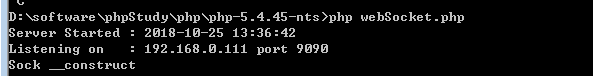• 页面: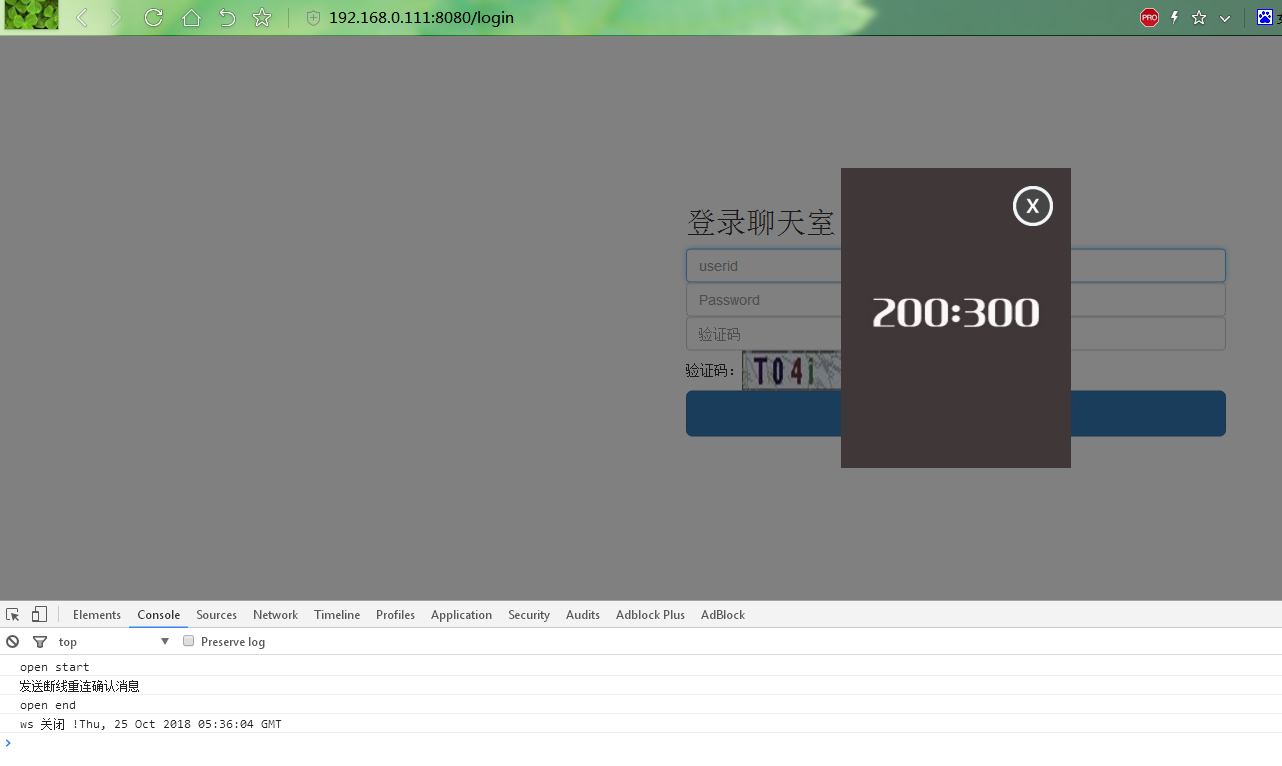展开全文• 我自己平时使用其他浏览器的时候都是使用广告屏蔽插件的，现在推荐给还没有使用过的小伙伴们，减少工作学习时无关信息的干扰。 目录ChromeFirefox Chrome Chrome中我一般使用的是Adblock Plus这个插件，这个插件不仅...

今天无意中打开MS Edge浏览网页时感觉广告好多，看起来很难受。我自己平时使用其他浏览器的时候都是使用广告屏蔽插件的，现在推荐给还没有使用过的小伙伴们，减少工作学习时无关信息的干扰。

# Chrome这个时候相应的插件列表就出现了，然后我们选择图标为如下所示的插件：点击右侧的Add to Chrome按钮就完成安装了。
安装好的插件的图标会出现在浏览器的右上角，点击图标后在弹出的对话框中我们可以选择打开或者关闭这个插件。

# Firefox

我在Firefox中经常使用的广告屏蔽插件是uBlock Origin。下面简单说一下它的安装过程。
如图所示，点击Firefox浏览器右上角的三条杠的图标。然后在弹出的选项卡中选择附加组件，继而在出现的页面中点击蓝色的查看更多附加组件按钮。这个又出现一个新的页面，我们在这个页面右上方的搜索框中输入uBlock Origin后回车，页面就跳转到对应的插件按钮了。插件的图标是这样的：然后点击+添加到Firefox按钮，这个时候会弹出一个对话框，如图所示：点击添加就完成了安装。
同样，在Firefox浏览器的右上角也会出现对应的插件按钮，点击该按钮可以选择打开或者关闭插件。
以上介绍的是我常用的两个插件，事实上还有很多其他可选择的插件，只是我用这两个插件有一年多了没有出现过问题，所以才推荐给大家。像Chrome和Firefox这样的浏览器插件用好了使用起来会很舒服，希望我的这篇小文能对小伙伴们有些许帮助。

展开全文Chrome Firefox
• 启用此扩展程序后，它将阻止打开新的选项卡或新窗口，尤其是那些用户不希望看到的选项卡或新窗口，以及导致不必要的广告的窗口。这将减少必须查找并关闭新打开的选项卡或窗口的烦恼，尤其是在屏幕右下角打开新窗口时...web javascript
• 厌倦了广告拦截程序传递的广告？想要为您的浏览器提供一个简单的可配置附件，它会自动为您关闭标签吗？那就别再看了！功能：-配置URL搜索模式以自动关闭标签-支持文本和正则表达式搜索-导入和导出配置这是显示示例...
• 这是用Kotlin编写的模块化音频广告拦截器的概念证明，具有现代且简单的用户界面。 产品特点 无需ROOT 播放广告时关闭声音 播放任意音频而不是广告 基于插件的设计 支持Spotify 对Soundcloud的支持（实验性） 使...
• 隐私设置与安全性但是我的高级设置里没有隐私设置与安全性，拦截网站的原因是安装了广告拦截器的插件 第一步：点击设置里的扩展程序 第二步：扩展程序的广告终结者–>详情 第三步：点击扩展程序选项 第四步： ...
• ## 谷歌屏蔽广告插件

千次阅读 2019-03-06 19:51:36
chrome屏蔽网页上关闭不了的广告插件 使用方法: 在谷歌浏览器上新建标签页，打开 chrome://extensions/ 将下载的.crx文件直接拖放到谷歌浏览器中，即可安装成功 转载: ...
• 这个开源扩展可以自动地阻止加密挖掘...在CoinHive发布适当的修复程序之前（例如，在开始挖掘之前需要获得所有访问者的同意），我建议手动向您喜欢的广告拦截器中添加一些屏蔽规则或安装此扩展程序。 支持语言:Englishchrome
• 网民在浏览网页时，总会被大量广告弹窗、垃圾信息、流氓行为所干扰，降低我们的网络体验；有时不小心点击到垃圾弹窗，电脑会自动植入一些木马病毒，降低电脑使用寿命；这时浏览器预处理就会显得很重要； 这里展示一...
• 通过启用浏览器自带的广告拦截功能，或为浏览器安装广告拦截插件，能够还原网页原本的清爽真容。浏览器自带-安卓广告拦截操作说明口碑比较好的 Via、夸克和 X浏览器都带有广告拦截功能。夸克浏览器貌似是默认开启的...
• 如何屏蔽搜狗浏览器中的广告 搜狗浏览器拦截广告的教程 2、点击扩展按钮会出现一个下拉菜单，点击【获取】 如何屏蔽搜狗浏览器中的广告 搜狗浏览器拦截广告的教程 3、弹出一个扩展商店 如何屏蔽搜狗浏览器中的广告 ...大数据
• 谷歌CSDN去广告插件，使用最新版谷歌扩展API v3，支持最新版本谷歌浏览器css javascript
• 至于js语法介绍，则太过于琐碎，我了解也不多，详情请点击这里 拦截广告实战 网页上的广告一般是站长在网页植入一段js代码，要想屏蔽广告只需要将这些js屏蔽掉即可。 下面示范如何使用chrome屏蔽广告，帮助我们更好...
• 前言 相信很多刚买电脑的小伙伴，新电脑用上几天就发现电脑上会时...1.关闭windows10上应用广告 2.关闭网页浏览器广告 3.删除一些流氓软件 1.关闭windows10上应用广告 由于系统原因win10系统会时不时向我们...windows
• 1.浏览器安装插件 Tampermonkey。 2.在Tampermonkey中运行自己的插件**.user.js 3.谷歌和360浏览器验证成功。
• 前言： ...因本人有强迫症，就是不喜欢软件给我弹各种“垃圾”，而且是隐蔽的，静默的，给我安装了各种无用的插件。 起因： &amp;nbsp;&amp;nbsp;&amp;nbsp;&amp;nbsp;&amp;nbsp;&aadblock
• title: Chrome-AdGuard广告拦截器 tags: 未定义 categories: 工具推荐 date: 2019-11-04 20:14:55 一款无与伦比的广告拦截扩展，对抗各式广告与弹窗。 AdGuard 广告拦截器可有效的拦截所有网页上的所有类型的...
• 3.广告拦截：能拦截所有广告，阻止弹出窗口； 4.页面静音：页面声音打开或者关闭自由控制，上网听音乐玩游戏随心所欲； 5.网页收藏夹：按照您的喜好来自定义自己的收藏夹； 6.历史记录：可以保留历史记录也可以......